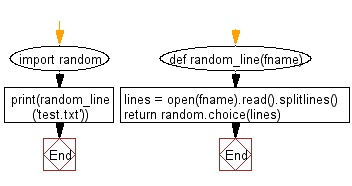﻿ Python File I/O: Read a random line from a file - w3resource# Python File I/O: Read a random line from a file

## Python File I/O: Exercise-15 with Solution

Write a Python program to read a random line from a file.

Sample Solution:-

Python Code:

``````import random
def random_line(fname):
return random.choice(lines)
print(random_line('test.txt'))
```
```

Sample Output:

```Append this text.
```

Flowchart:Python Code Editor:

Have another way to solve this solution? Contribute your code (and comments) through Disqus.

What is the difficulty level of this exercise?

Test your Python skills with w3resource's quiz

﻿

## Python: Tips of the Day

Merges two or more lists into a list of lists, combining elements from each of the input lists based on their positions

Example:

```def tips_merge(*args, fill_value=None):
max_length = max([len(lst) for lst in args])
result = []
for i in range(max_length):
result.append([
args[k][i] if i < len(args[k])
else fill_value for k in range(len(args))
])
return result
print(tips_merge(['x', 'y'], [1, 2], [True, False]))
print(tips_merge(['x'], [1, 2], [True, False]))
print(tips_merge(['x'], [1, 2], [True, False], fill_value = '_'))
```

Output:

```[['x', 1, True], ['y', 2, False]]
[['x', 1, True], [None, 2, False]]
[['x', 1, True], ['_', 2, False]]
```